085-1263404

### My Journal

All things Mathematical
10 Dec 2018

## Question 1

A racing car covers a journey of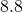km from rest to rest. It accelerates uniformly in the first minute to reach its maximum speed of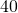m/s, it holds this speed for a certain time and then slows uniformly to rest with a retardation of magnitude three times that of the acceleration.

i) Draw a rough velocity-time graph and find the distance travelled in the three stages of the journey and the total time taken.

ii) If the maximum speed over the final kilometre of the journey had been restricted to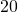m/s, show that the time taken from rest to rest would have been at least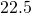s longer than before, assuming the same rates of acceleration and deceleration as before.

[Video Solution]

## Question 2

From a point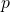on horizontal ground an elastic particle is projected under gravity with a velocity of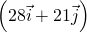m/s, where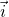and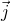are unit vectors along the horizontal and upward drawn vertical, respectively.

i) Find the displacement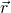fromat timeseconds afterwards, and in particular when the particle is at its highest point.

ii) If at this point [the highest point] the particle strikes a fixed vertical wall, where the coefficient of restitution is, find how far fromthe particle strikes the ground.

[Video Solution]

## Question 3

a) State the laws governing oblique, perfectly elastic collision between two spheres.

[Video Solution]

b) A small sphere collides obliquely with a similar sphere of equal mass at rest – both spheres being smooth and perfectly elastic.

i) Show that the paths of the two spheres after the collision are at right angles.

ii) Prove that there is no loss in kinetic energy.

[Video Solution]

## Question 4

A wedge of mass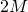rests on a smooth horizontal table with one of its plane faces inclined at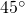to the horizontal. This plane face is smooth and on it is placed a particle of mass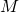and the system is released from rest.

i) Draw separate diagrams showing the forces acting on the particle and on the wedge during the motion.

ii) By considering the acceleration of the particle in two components, one component down the plane and the other horizontal, show that the acceleration of the wedge is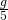and that of the particle is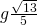.

[Video Solution]

## Question 5

a) Using the usual notation, prove that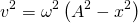represents simple harmonic motion.

[Video Solution]

b) A light flexible elastic string of natural length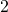m and elastic constant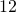N/m has one endtied to a point on a smooth horizontal table. To its other end is attached a particle of mass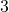kg, which is placed on the table at a point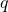, distance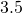m fromand released from rest. Show that in the first stage of motion the particle moves with simple harmonic motion of period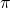seconds and that the time taken to reachis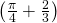s.

[Video Solution]

## Question 6

a) Show that a particle moving in a circle with constant speed is being accelerated towards the centre of the circle.

[Video Solution]

b) A small ring of masskg is threaded on a smooth light flexible inelastic string of length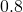m. The ends of the string are attached to two fixed points, distance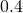m apart in the same vertical line, and the ring describes with constant speed a horizontal circle whose centre is the lower fixed point. Find the constant speed of rotation and show that the tension in the string is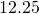N.

[Video Solution]

## Question 7

A uniform rod of length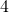m and weight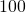N is smoothly hinged at one end to a rough horizontal floor. The rod rests on the smooth curved surface of a hemisphere whose plane face is on the floor. The rod is in equilibrium inclined atto the horizontal and the hemisphere, of weight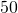N and radiusm, is in limiting equilibrium.

i) Show in separate diagrams the forces acting on the rod and on the hemisphere.

ii) Find the reaction between the rod and the hemisphere,

iii) and prove that the coefficient of friction between the latter and the floor is.

[Video Solution]

## Question 8

a) Prove that the moment of inertia of a uniform rod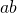of massand length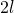about an axis through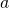, perpendicular to the rod, is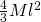.

[Video Solution]

b i) Such a rod [from part (a)] is free to rotate in a vertical plane about a fixed horizontal axis at, with a particle of massattached to the rod at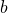. The system is released from rest with the rod vertical and the endabove. Show that the angular speed of the rod when it is next vertical is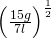.

ii) At this point the particle falls off. Find the height to which the endsubsequently rises.

[Video Solution]

## Question 9

A particle of masskg is projected vertically upwards from ground level with a speed of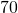m/s. In addition to the weight of the particle, there is the resistance force of the air of magnitude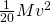newtons when the speed if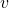metres per second. Show that the equation of motion during the upward journey is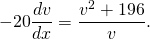Prove that the maximum height is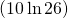m, and that the time taken to reach it is approximately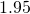s.

(Note: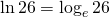)

[Video Solution]

## Question 10

a) State the condition for the equilibrium of a floating body.

[Video Solution]

b) A uniform rectangular board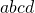of weight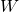, floats with the diagonal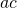on the surface of the of the water, the lowest cornerbeing attached to the bottom of the vessel by a light inelastic string.

i) Show in a diagram the forces acting on the board and prove that the specific gravity of the board is.

ii) Find the tension in string.

[Video Solution]

### Latest PSI Licence:

“Contains Irish Public Sector Information licensed under a Creative Commons Attribution 4.0 International (CC BY 4.0) licence”.

### Important Exception to the above Licence:

The State Examination Commission is the copyright holder which is providing the material under the above license (as per current directives and regulations from the relevant government bodies). However the State Examination Commission as an Irish examination body is able to use copyrighted material in its exams without infringing copyright but this right is not extended to third parties when those exams are re-used.

(For example: the State Examination Commission may include in their exam a copyrighted poem and this action does not require the permission of the poet but the poet’s permission must be sought when the exam is re-used by someone other than the State Examination Commission.)

Also, all derived and related work (such as video solutions, lessons, notes etc) are the copyrighted material of Stephen Easley-Walsh (unless stated otherwise). And that the above licence is for only the exam itself and nothing further.

### Citation:

State Examinations Commission (2018). State Examination Commission. Accessed at: https://www.examinations.ie/?l=en&mc=au&sc=ru

Malone, D and Murray, H. (2016). Archive of Maths State Exams Papers. Accessed at: http://archive.maths.nuim.ie/staff/dmalone/StateExamPapers/

This site uses Akismet to reduce spam. Learn how your comment data is processed.